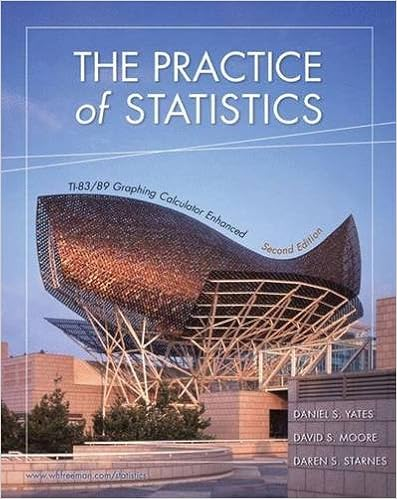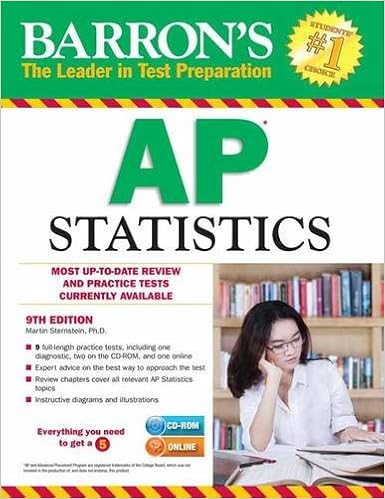## Long and Synthesis Division

After many months of SAT preparation and the first SAT exam was finished, our Math class tilts toward precalculus. We studied the function’s behavior, transformation, and shapes. Many times, we find zeros of polynomials through couple strategies such as Descartes’s rule of Signs, Upper and Lower Bounds for zero, list of possible zeros (factor of constant term/factor of leading coefficient), factoring and long division and synthetic division.

I found out that long division and synthetic division to solve for zeros is really essential, so I want to show how to use them.

1. Long Division

It looks almost the same with normal division we did in grade 2. However, complexity but the same element.2.  Synthetic division

The same problem as above, the synthetic division has a different way but result in the same answer.## Preparing for SAT exam

As the SAT exam is approaching, in Math class I have been preparing and practicing taking the exams. In the math sections of the exam will cover algebra, equations, proportion, statistics and some geometry content. Most of the problems I could be able to solve them, however, due to the time limit I must solve the questions fast enough with accuracy. I will share one of the strategies we have been gone over to solve a specific type of questions: special pattern circles.

## AP Statistics and AP Computer Science A scores

Two more years till my graduation, I might attend a university oversea, therefore to prepare for university, I took two AP (Advanced Placement) courses, AP Statistics and AP Computer Science A. The courses are university-level course and they are extremely difficult. To prepare for AP exams on May, I studied statistics during Math class and part time in school prepare for AP computer science.

This is my score for the AP course:

AP Statistics: 4

AP Computer Science: 4

The final reported on 5-points scale as follow:

5 = extremely qualified

4 = well qualified

3 = qualified

2 = possible qualified

1 = no recommendation.

## AP Statistics

Arthur Benjamin, an American mathematician quoted that

Teach statistics before calculus!

Most students, after they finished learning Algebra they will learn calculus but not statistic. Though, calculus only use in scientific researches but statistic is what most people would need in their daily life. People saw a lot of data, graphs, scatter plots in articles, documents or in news and statistic will teach you how to analyze them.

Now, I’m studying AP Statistics which is a college level course and I’m preparing for coming up exam. This course is very challenging due to many new and advanced vocabularies and it requires a lot of understanding and analyzing. However, learning about statistics changed my perspective, how I see data and examine about them if they were valid. Through analyzing and constructing bar graphs and scatter plot and I found out that it is actually fun.

We study based on The Practice of Statistics Textbook, AP statistics test book. Other than this, we learn and practice from many other online resource such as Khan Academy and other worksheets.After finishing one chapter, we have to take a reviewing quiz before continue the next one.

Currently, we finished four chapters:

Chapter 1: Exploring Data

Chapter 2: The normal Distribution

Chapter 3: Examining Relationship

Chapter 4: More on Two-Variable Data